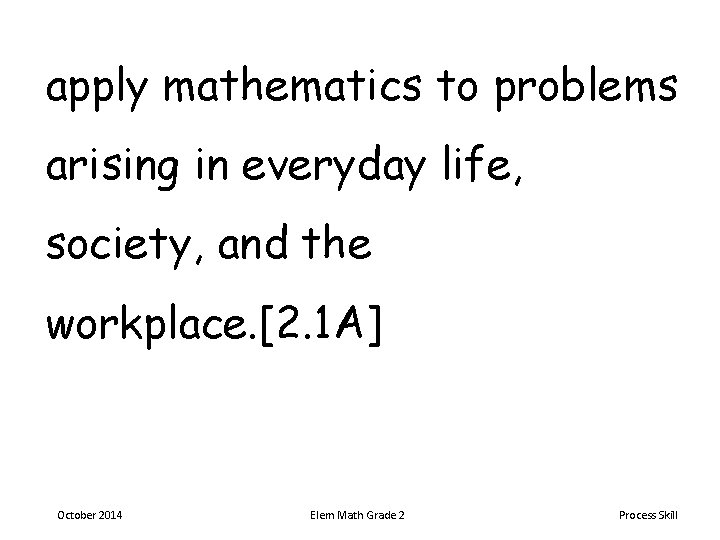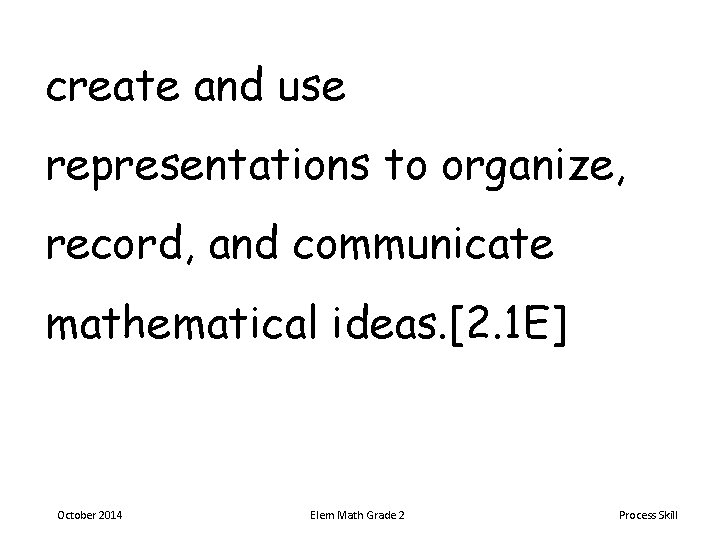# apply mathematics to problems arising in everyday life

• Slides: 7apply mathematics to problems arising in everyday life, society, and the workplace. [2. 1 A] October 2014 Elem Math Grade 2 Process Skilluse a problem-solving model that incorporates analyzing given information, formulating a plan or strategy, determining a solution, justifying the solution, and evaluating the problem-solving process and the reasonableness of the solution. [2. 1 B] October 2014 Elem Math Grade 2 Process Skillselect tools, including real objects, manipulatives, paper and pencil, and technology as appropriate, and techniques, including mental math, estimation, and number sense as appropriate, to solve problems. [2. 1 C] October 2014 Elem Math Grade 2 Process Skillcommunicate mathematical ideas, reasoning, and their implications using multiple representations, including symbols, diagrams, graphs, and language as appropriate. [2. 1 D] October 2014 Elem Math Grade 2 Process Skillcreate and use representations to organize, record, and communicate mathematical ideas. [2. 1 E] October 2014 Elem Math Grade 2 Process Skillanalyze mathematical relationships to connect and communicate mathematical ideas. [2. 1 F] October 2014 Elem Math Grade 2 Process Skilldisplay, explain, and justify mathematical ideas and arguments using precise mathematical language in written or oral communication. [2. 1 G] October 2014 Elem Math Grade 2 Process Skill